Open in App
Not now

# Stripplot using Seaborn in Python

• Difficulty Level : Medium
• Last Updated : 06 Nov, 2020

Seaborn is an amazing visualization library for statistical graphics plotting in Python. It provides beautiful default styles and color palettes to make statistical plots more attractive. It is built on top of the matplotlib library and also closely integrated into the data structures from pandas.

### Strip plot

A strip plot is drawn on its own. It is a good complement to a boxplot or violinplot in cases where all observations are shown along with some representation of the underlying distribution. It is used to draw a scatter plot based on the category.

Syntax: seaborn.stripplot(*, x=None, y=None, hue=None, data=None, order=None, hue_order=None, jitter=True, dodge=False, orient=None, color=None, palette=None, size=5, edgecolor=’gray’, linewidth=0, ax=None, **kwargs)

Parameters:

• x, y, hue: Inputs for plotting long-form data.
• data: Dataset for plotting.
• order: It is the order to plot the categorical levels in.
• color: It is the color for all of the elements, or seed for a gradient palette

Returns: This method returns the Axes object with the plot drawn onto it.

Example: Basic visualization of “tips” dataset using stripplot()

## Python3

 `import` `seaborn ` `import` `matplotlib.pyplot as plt ` `      `  `seaborn.``set``(style ``=` `'whitegrid'``)   ` `tip ``=` `seaborn.load_dataset(``"tips"``)   ` `      `  `seaborn.stripplot(x``=``"day"``, y``=``"total_bill"``, data``=``tip) ` ` `  `plt.show() `

Output: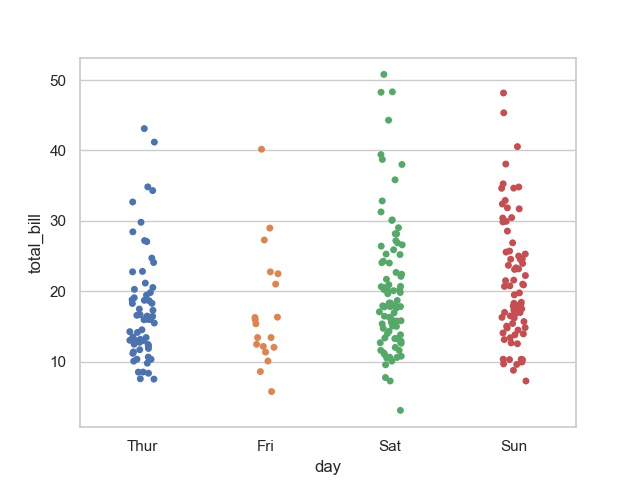## Draw a single horizontal strip plot using Stripplot

If we use only one data variable instead of two data variables then it means that the axis denotes each of these data variables as an axis.

X denotes an x-axis and y denote a y-axis.

Syntax:

`seaborn.stripplot(x)`

Code:

## Python3

 `# Python program to illustrate ` `# Stripplot using inbuilt data-set ` `# given in seaborn ` ` `  `# importing the required module ` `import` `seaborn ` `import` `matplotlib.pyplot as plt ` ` `  `# use to set style of background of plot ` `seaborn.``set``(style ``=` `'whitegrid'``) ` ` `  `# loading data-set ` `tips ``=` `seaborn.load_dataset(``"tips"``) ` `seaborn.stripplot(x``=``tips[``"total_bill"``]) `

Output: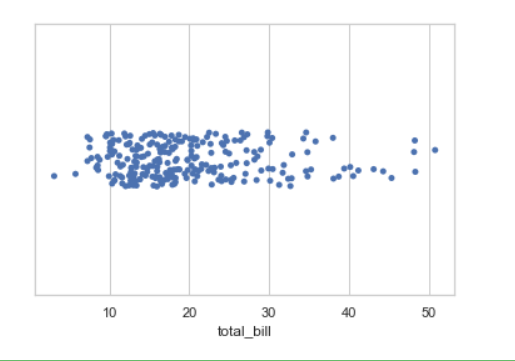## Draw strip plot using jitter parameter

jitter can be used to provide displacements along the horizontal axis, which is useful when there are large clusters of data points. You can specify the amount of jitter (half the width of the uniform random variable support), or just use True for a good default.

Syntax:

seaborn.stripplot(x, y, data, jitter)

Code:

## Python3

 `# Python program to illustrate ` `# Stripplot using inbuilt data-set ` `# given in seaborn ` ` `  `# importing the required module ` `import` `seaborn ` `import` `matplotlib.pyplot as plt ` ` `  `# use to set style of background of plot ` `seaborn.``set``(style ``=` `'whitegrid'``) ` ` `  `# loading data-set ` `tips ``=` `seaborn.load_dataset(``"tips"``) ` ` `  `seaborn.stripplot(x``=``"day"``, y``=``"total_bill"``, data``=``tips, jitter``=``0.1``)`

Output: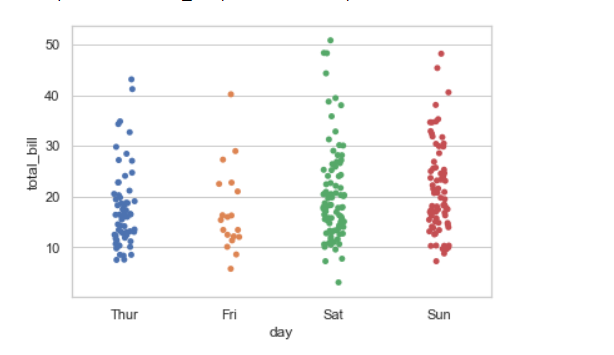## Draw outlines around the data points using linewidth

Width of the gray lines that frame the plot elements. Whenever we increase linewidth than the point also will increase automatically.

Syntax:

seaborn.stripplot(x, y, data, linewidth)

## Python3

 `# Python program to illustrate ` `# Stripplot using inbuilt data-set ` `# given in seaborn ` ` `  `# importing the required module ` `import` `seaborn ` `import` `matplotlib.pyplot as plt ` ` `  `# use to set style of background of plot ` `seaborn.``set``(style ``=` `'whitegrid'``) ` ` `  `# loading data-set ` `tips ``=` `seaborn.load_dataset(``"tips"``) ` ` `  `seaborn.stripplot(y``=``"total_bill"``, x``=``"day"``, data``=``tips, ` `                   ``linewidth``=``3``)`

Output: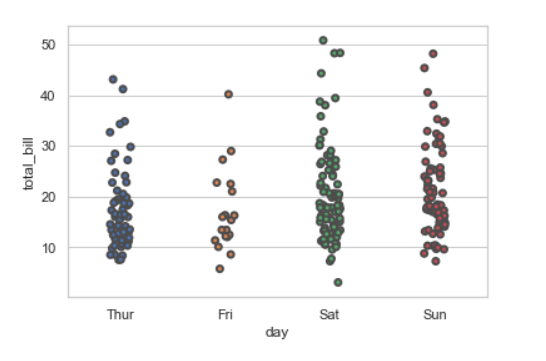We can change the color with edgecolor

## Python3

 `seaborn.stripplot(y``=``"total_bill"``, x``=``"day"``, data``=``tips, ` `                   ``linewidth``=``2``,edgecolor``=``'green'``)`

Output: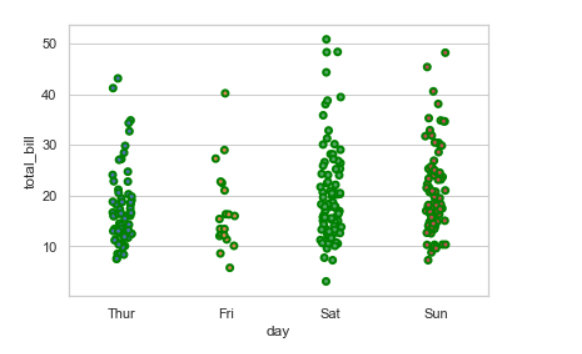## Draw strip plot using hue parameter

While the points are plotted in two dimensions, another dimension can be added to the plot by coloring the points according to a third variable.

Syntax:

sns.stripplot(x, y, hue, data);

## Python3

 `# Python program to illustrate ` `# Stripplot using inbuilt data-set ` `# given in seaborn ` ` `  `# importing the required module ` `import` `seaborn ` `import` `matplotlib.pyplot as plt ` ` `  `# use to set style of background of plot ` `seaborn.``set``(style ``=` `'whitegrid'``) ` ` `  `# loading data-set ` `tips ``=` `seaborn.load_dataset(``"tips"``) ` ` `  `seaborn.stripplot(x``=``"sex"``, y``=``"total_bill"``, hue``=``"day"``, data``=``tips)`

Output: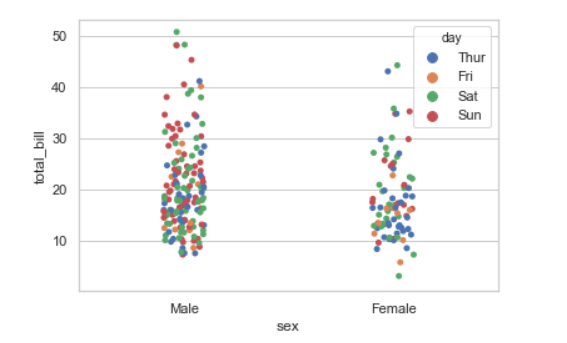### Draw each level of the hue variable at different locations on the major categorical axis

When using hue nesting, setting dodge should be True will separate the strips for different hue levels along the categorical axis. And Palette is used for the different levels of the hue variable.

Syntax:

seaborn.stripplot(x, y, data, hue, palette, dodge)

## Python3

 `seaborn.stripplot(x``=``"day"``, y``=``"total_bill"``, hue``=``"smoker"``, ` `                   ``data``=``tips, palette``=``"Set2"``, dodge``=``True``)`

Output: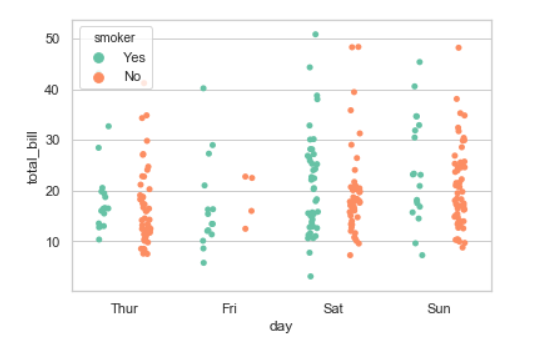Possible values of palette are :

Accent, Accent_r, Blues, Blues_r, BrBG, BrBG_r, BuGn, BuGn_r, BuPu, BuPu_r,

CMRmap, CMRmap_r, Dark2, Dark2_r, GnBu, GnBu_r, Greens, Greens_r, Greys, Greys_r,

OrRd, OrRd_r, Oranges, Oranges_r, PRGn, PRGn_r, Paired, Paired_r, Pastel1, Pastel1_r, Pastel2,

Pastel2_r, PiYG, PiYG_r, PuBu, PuBuGn, PuBuGn_r, PuBu_r, PuOr, PuOr_r, PuRd, PuRd_r, Purples,

Purples_r, RdBu, RdBu_r, RdGy, RdGy_r, RdPu, RdPu_r, RdYlBu, RdYlBu_r, RdYlGn, RdYlGn_r, Reds,

Reds_r, Set1, Set1_r, Set2, Set2_r, Set3, Set3_r, Spectral, Spectral_r, Wistia, Wistia_r, YlGn,

YlGnBu, YlGnBu_r, YlGn_r, YlOrBr, YlOrBr_r, YlOrRd, YlOrRd_r, afmhot, afmhot_r, autumn, autumn_r,

binary, binary_r, bone, bone_r, brg, brg_r, bwr, bwr_r, cividis, cividis_r, cool, cool_r, coolwarm,

coolwarm_r, copper, copper_r, cubehelix, cubehelix_r, flag, flag_r, gist_earth, gist_earth_r,

gist_gray, gist_gray_r, gist_heat, gist_heat_r, gist_ncar, gist_ncar_r, gist_rainbow, gist_rainbow_r,

gist_stern, gist_stern_r, gist_yarg, gist_yarg_r, gnuplot, gnuplot2, gnuplot2_r, gnuplot_r,

gray, gray_r, hot, hot_r, hsv, hsv_r, icefire, icefire_r, inferno, inferno_r, jet, jet_r, magma,

## Plotting large points and different aesthetics With marker and alpha parameter

We will use alpha to manage transparency of the data point, and use marker for marker to customize the data point.

## Python3

 `import` `seaborn ` `import` `matplotlib.pyplot as plt ` `      `  `seaborn.``set``(style ``=` `'whitegrid'``) ` ` `  `tips ``=` `seaborn.load_dataset(``"tips"``) ` ` `  `seaborn.stripplot(x``=``"day"``, y``=``"total_bill"``, hue``=``"smoker"``, ` `                  ``data``=``tips, palette``=``"Set1"``, size``=``20``, ` `                  ``marker``=``"s"``, alpha``=``0.2``) ` ` `  `plt.show() `

Output: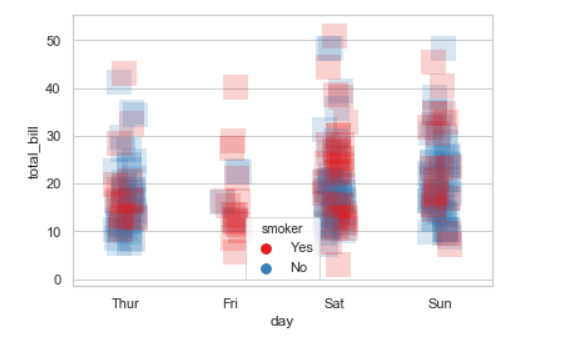My Personal Notes arrow_drop_up
Related Articles